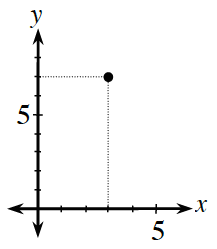### Home > CC3MN > Chapter 5 Unit 6 > Lesson INT1: 5.1.1 > Problem5-11

5-11.

Find the rule for the pattern represented below.

 Tile Tile Tile Tile Tile Tile Tile Tile Tile Tile Tile

Figure 1Create a table to help find a pattern.

Fill in the information that you are given.

What is the difference between the number of tiles in the two figures?

Use this information to find the difference between the number of tiles in consecutive figures.

Now fill in the rest of the table.

 Figure # # of tiles

 Figure # $\color{darkorange}{1}$ $\color{darkorange}{3}$ # of tiles $\color{darkorange}{11}$ $\color{darkorange}{7}$ $\quad \boldsymbol{\uparrow} {} \\ \text{From} \\ \text{figure}$ $\quad \boldsymbol{\uparrow} {} \\ \text{From} \\ \text{graph}$

 Figure # $\color{darkorange}{1}$ $\color{darkorange}{3}$ # of tiles $\color{darkorange}{11}$ $\color{darkorange}{7}$ $\quad \boldsymbol{\uparrow} {} \\ \text{From} \\ \text{figure}$ $\color{blue}{-4}$ $\quad \boldsymbol{\uparrow} {} \\ \text{From} \\ \text{graph}$

 Figure # $\color{darkorange}{1}$ $\color{darkorange}{3}$ # of tiles $\color{darkorange}{11}$ $\color{darkorange}{7}$ $\quad\color{turquoise}{-2}\ \quad \boldsymbol{\uparrow} \ \color{turquoise}{-2} \\ \quad \quad \ \ \ \text{From} \\ \quad \quad \ \ \ \text{figure}$ $\color{turquoise}{-2}\ \ \boldsymbol{\uparrow} \ \color{turquoise}{-2} \\ \quad\text{From} \\ \quad\text{graph}$

 Figure # $\color{purple}{0}$ $\color{darkorange}{1}$ $\color{purple}{2}$ $\color{darkorange}{3}$ $\color{purple}{4}$ # of tiles $\color{purple}{13}$ $\color{darkorange}{11}$ $\color{purple}{9}$ $\color{darkorange}{7}$ $\color{purple}{5}$ $\quad\color{turquoise}{-2}\ \quad \boldsymbol{\uparrow} \ \color{turquoise}{-2} \\ \quad \quad \ \ \ \text{From} \\ \quad \quad \ \ \ \text{figure}$ $\color{turquoise}{-2}\ \ \boldsymbol{\uparrow} \ \color{turquoise}{-2} \\ \quad\text{From} \\ \quad\text{graph}$

 Figure # $\color{purple}{0}$ $\color{darkorange}{1}$ $\color{purple}{2}$ $\color{darkorange}{3}$ $\color{purple}{4}$ # of tiles $\enclose{circle}{\color{purple}{13}}$ $\color{darkorange}{11}$ $\color{purple}{9}$ $\color{darkorange}{7}$ $\color{purple}{5}$ $\quad\color{turquoise}{-2}\ \quad \boldsymbol{\uparrow} \ \color{turquoise}{-2} \\ \quad \quad \ \ \ \text{From} \\ \quad \quad \ \ \ \text{figure}$ $\enclose{circle}{\color{turquoise}{-2}}\ \ \boldsymbol{\uparrow} \ \color{turquoise}{-2} \\ \quad\text{From} \\ \quad\text{graph}$

Using the starting point and the growth rate, write an equation to represent the pattern.